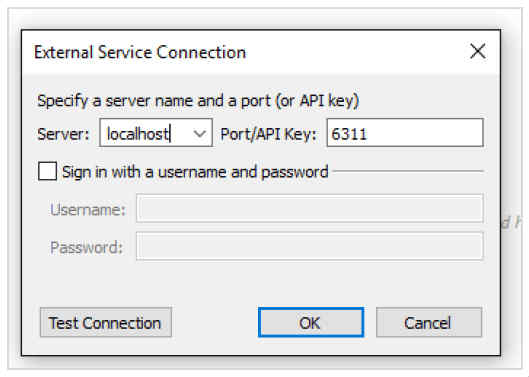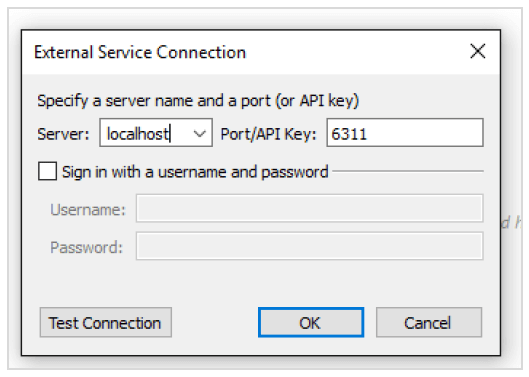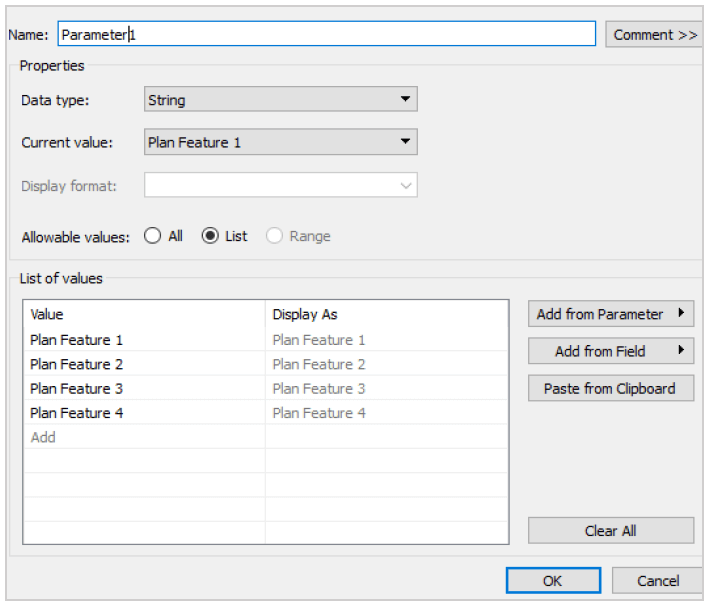# Random Forest in Tableau using R

Random Forest in Tableau using R

I have been using Tableau for some time to explore and visualize the data in a beautiful and meaningful way. Quite recently, I have learned that there is a way to connect Tableau with R-language, an open source environment for advanced Statistical analysis. Marrying data mining and analytical capabilities of R with the user-friendly visualizations of Tableau would give us the ability to view and optimize the models in real-time with a few clicks.

As soon as I discovered this, I tried to run the machine learning algorithm Random forest from Tableau. Random forest is a machine learning technique to identify features (independent variables) that are more discerning than others in explaining changes in a dependent variable. It achieves that by ensembling multiple decision trees that are constructed by randomizing the combination and order of variables used.

The prediction accuracy of Random forest depends on the set of explanatory variables used in the formula. To arrive at the set of variables that makes the best prediction, one often needs to try multiple combinations of explanatory variables and then analyze the results to assess the accuracy of the model. Connecting R with Tableau will help you save a lot of time that would have otherwise gone into the tedious task of importing the data into Tableau every time you add/remove a variable.

Tableau has a function script_real() that lets you run R-scripts from Tableau. To use this function in any calculated field, you need to set up the connection by following steps:

1. Open R Studio and install the package ‘Rserve’

install.packages(“Rserve”)

2. Run the function Rserve()

library(Rserve)
Rserve()

3. Once you see the message “Starting Rserve…”, open tableau and follow the below steps to setup the connectionWhen you click on “Manage External Service Connection” or “Manage R Connection” depending on the version of Tableau, you’ll see the following window.Click OK to complete the connection between Tableau and R on your machine.

Let’s take a simple example to understand how to leverage the connection with R to run Random Forest. In this example, I need to predict the enrollments for an insurance plan based on its features (say costs and benefits) and the past performance of similar plans.

After importing the dataset into Tableau, we need to create a calculated field using the function script_real() to run the script for random forest which looks like below:

library(randomForest)
Data15 Data16 attach(Data15)
formula<-Enrollments~
Plan.feature.1+
Plan.feature.2+
Plan.feature.3+
Plan.feature.4
rf ntree= 1000, Importance = TRUE, do.trace = 100,
na.action=na.omit)
yhat Data16\$Enrollments<-yhat
testdata<-rbind(Data15,Data16)

To run the same script in Tableau using the function script_real(), we need to create a dataframe using only the required columns in the imported dataset. This should be done using the arguments .arg1…arg5 instead of actual column names since R will be able to access only the data that’s referred through arguments.

The values for these arguments should be passed at the end of the R-script in the respective order i.e., .arg1 will take the values of the first mentioned field, .arg2 will take the values of the second mentioned field and so on.

After making these changes, the code will look like the following:

Script_real(
‘library(randomForest)
Data=data.frame(.arg1, .arg2, .arg3, .arg4, .arg5, .arg6)
Data15 Data16 formula<-.arg2~.arg3+.arg4+.arg5+.arg6
rf ntree= 1000, Importance = TRUE, do.trace = 100, na.action=na.omit)
yhat Data16\$.arg2<-yhat
testdata<-rbind(Data15, Data16)
testdata\$.arg2′, ATTR([Year]),SUM([Enrollments]), SUM([Plan feature 1]),SUM([Plan feature 2]),SUM([Plan feature 3]),SUM([Plan feature 4]))

The calculation must be set to “Plan ID” level to get the predictions for each plan ID.

Although this approach achieves the objective of predicting enrollments for each plan, it doesn’t offer us the flexibility to run multiple iterations without having to change the code manually. To make the model running easier, we can create parameters as shown below to choose the variables that go into the model.Then, we can create calculated fields (as shown below) whose values change based on the variables selected in the parameters.

case [Parameter1]
when “Plan Feature 1” then [Plan feature 1]
when “Plan Feature 2” then [Plan feature 2]
when “Plan Feature 3” then [Plan feature 3]
when “Plan Feature 4” then [Plan feature 4]
ELSE 0
END

After replacing the variables in code with parameters, the code will look like below:

Script_real(
‘library(randomForest)
Data=data.frame(.arg1, .arg2, .arg3, .arg4, .arg5, .arg6)
Data15 Data16 formula<-.arg2~.arg3+.arg4+.arg5+.arg6
rf ntree= 1000, Importance = TRUE, do.trace = 100, na.action=na.omit)
yhat Data16\$.arg2<-yhat
testdata<-rbind(Data15, Data16)
testdata\$.arg2′, ATTR([Year]),SUM([Enrollments]), SUM([var 1]),SUM([var 2]),SUM([var 3]),
SUM([var 4]))

This will let us run multiple iterations of random forest very easily as compared to manually adding and deleting variables in the code in R for every iteration. But, as you might have observed, this code takes exactly 4 variables only. This might be a problem since having a fixed number of variables in the model is a privilege you rarely (read as never) have.

To keep the number of variables dynamic, a simple way in this case is to select “None” in the parameter which will make the corresponding variable 0 in the data. Random forest will ignore a column in the data if all the values are zero.

As long as the no of variables is not too high, you can create as many parameters and select “None” in the parameters when you don’t want to select any more variables.

### Related Post##### Text Mining & its Applications##### Python in Data Science##### Internet of Things Analytics